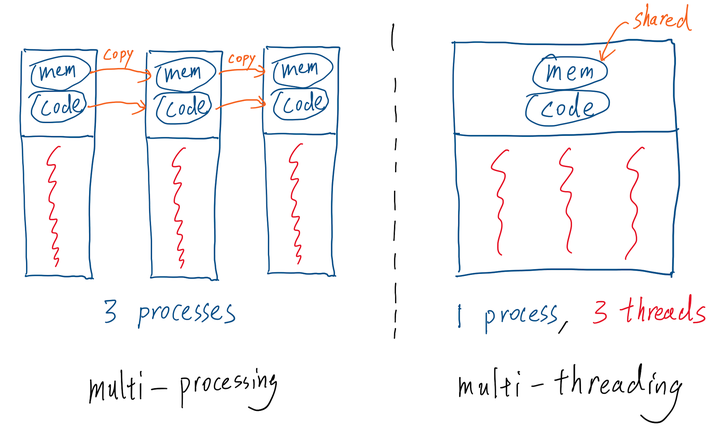# Multithreading in Python/PyTorch Using C++ ExtensionDespite having a built-in `threading` module, Python cannot do real multi-threading because of its infamous Global Interpreter Lock (GIL) mechanism.

Long story short, the GIL maintains a lock on the Python interpreter such that only one thread can use the interpreter at a time. As a result, any (CPU-bound) multithreading program in Python is actually executed in a single-threaded manner, or likely even slower, because of the overhead of maintaining multiple threads.

An alternative to achieve parallelism in Python is to use the Python built-in `multiprocessing` module. However, multi-processing is usually much heavier than multi-threading.

Process vs. Thread: To recap on the differences between process and thread, see the above figure. A process can have one or multiple threads. The main difference is that threads share virtual memory, program machine code and file descriptors; processes do not. This means spawning multiple processes using the `multiprocessing` package would require copying these overhead. Imagine we have a big tensor in memory on which we need to do some parallelizable computations. Using `multiprocessing` would make the program copy that big tensor many times into each spawned sub-processes and thus causing significant decrease in performance. Depending on the number of sub-processes spawned and the complexity of the parallelizable computation, this could cause our program to be slower than the original single-threaded implementation.

The solution: Port the parallelizable computation code to C++ and use the C++ `<thread>` standard library.

Step 1: Port code to C++: Writing code in C++ may sounds like a daunting task for many Python/PyTorch users. I felt the same when I first heard about it. But it turned out to be much easier than I thought was. We don’t need to write any complex Makefiles and all PyTorch tensor operations have a mapping in C++. In fact, the entire backbone of PyTorch is built with a C++ tensor library called `ATen`. PyTorch provide this tutorial on extending PyTorch code with C++. Some other useful documentations:

An side benefit of porting to C++ is that you get a speed-up even for just the literal translation, single-threaded implementation of your PyTorch equivalent code. This is because Python is just a slower language than C++ in general. You can read this article to learn more on why Python is slow. But to give you a preview, some of the main reasons include Python bing an interpreted language and Python being dynamically typed.

Step 2: Add parallelism to the C++ code: This tutorial gives a simple and clear introduction to the C++ `<thread>` library. The following example is copied from the tutorial. It shows 3 ways of constructing the thread object: using function pointer, using function object and using lambda expression.

``````// CPP program to demonstrate multithreading
// using three different callables.
#include <iostream>
using namespace std;

// A dummy function
void foo(int Z)
{
for (int i = 0; i < Z; i++) {
" pointer as callable\n";
}
}

// A callable object
public:
void operator()(int x)
{
for (int i = 0; i < x; i++)
" object as  callable\n";
}
};

int main()
{
cout << "Threads 1 and 2 and 3 "
"operating independently" << endl;

// This thread is launched by using
// function pointer as callable

// This thread is launched by using
// function object as callable

// Define a Lambda Expression
auto f = [](int x) {
for (int i = 0; i < x; i++)
" expression as callable\n";
};

// This thread is launched by using
// lamda expression as callable

// Wait for the threads to finish
// Wait for thread t1 to finish
th1.join();

// Wait for thread t2 to finish
th2.join();

// Wait for thread t3 to finish
th3.join();

return 0;
}

``````

Thread Pool: If your computation could use more threads than number of physical cores present in the machine, it would be wise to use a thread pool to re-use the threads. Recall that creating a thread has certain overhead, re-using existing threads can help alleviate this problem. Surprisingly, there has not been a official C++ thread pool implementation in standard library. But this simple and popular implementation on GitHub: `progschj/ThreadPool` works well. Simply download the `ThreadPool.h` header file and include it in your source file.

Here is an example usage, copied from the repo:

``````#include <iostream>
#include <vector>
#include <chrono>

int main()
{

std::vector< std::future<int> > results;

for(int i = 0; i < 8; ++i) {
results.emplace_back(
pool.enqueue([i] {
std::cout << "hello " << i << std::endl;
std::cout << "world " << i << std::endl;
return i*i;
})
);
}

for(auto && result: results)
std::cout << result.get() << ' ';  // this would wait until the repsective item in result is ready before returning
std::cout << std::endl;

return 0;
}
``````

Here, the `pool.enqueue()` is just like the `thread()` constructor, it can take either a function pointer, a function object or a lambda expression. The `emplace_back()` call would construct and append a `future` object as a placeholder to the return value of the thread task. A `future` object is a asynchronous mechanism: its constructor will always return immediately; the thread task will be running in its designated thread; only when we call `get()` on a `future` object, will the program actually wait for the task to finish and the result to be ready. Therefore, the `for` loop at the end effectively serves as a `join()` call to all running threads.